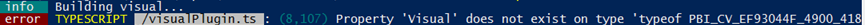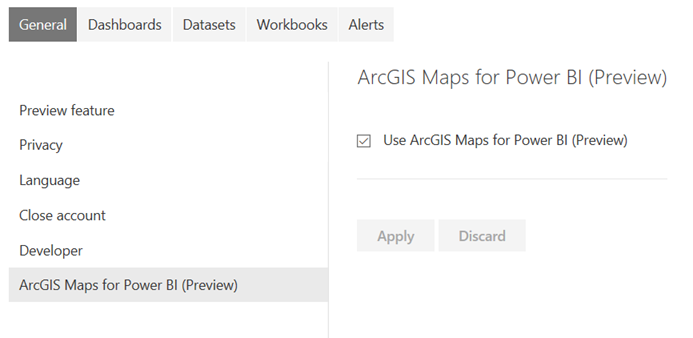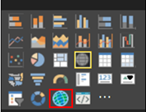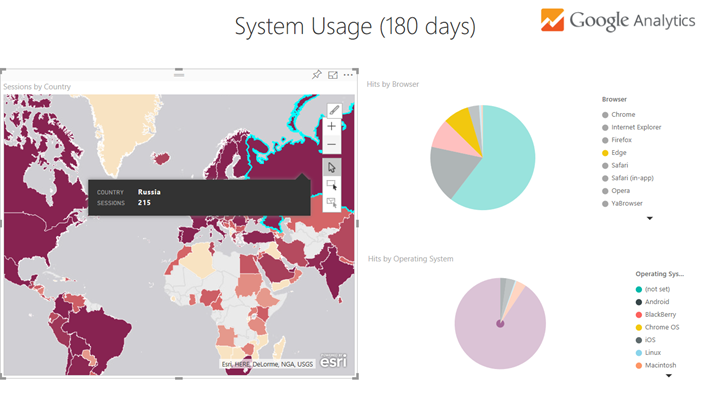# Tag Archives

50 Articles

## Updated: Annual radio countdown Top 2000 in Power BI

A bit later this year, but even after my move to the US I decided to keep up the tradition of updating my Power BI analysis of the Top 2000 analysis for this year.

Full screen

Enjoy and happy holidays! See you next year.

## Ultimate Time Based Calculations Cheat Sheet for DAX / Power BI (including Week based calculations)

Power BI provides great time intelligence features to calculate Year-to-date (YTD), Month-to-date (MTD) and Quarter-to-date (QTD) totals. There is no such thing as Week-to-date (WTD) or Period-to-date (PTD) where period could be any arbitrary period definition (I used two-month periods in my example below). If you want those, you will have to create the calculations yourself. I was inspired by this excellent blog post and created an ultimate time-intelligence calculations Power BI file. I used Matt Massons excellent date dimension generation script to generate the date table for my example.

Basic Measures

• Total Amount:
`TotalAmount = SUM(Sales[Amount])`
• Total Quantity:
`TotalQuantity = SUM(Sales[Quantity])`

Day Measures

• Total Amount for Last Day (Yesterday)
`Amount_LastDay = CALCULATE([TotalAmount],PREVIOUSDAY('Date'[Date]))`
• Total Amount for same Day last Year
`Amount_SameDayLastYear = CALCULATE([TotalAmount],SAMEPERIODLASTYEAR('Date'[Date]))`
• Variance of total Amount compared to Total Amount for Last Day
`Amount_DOD_Variance = [TotalAmount]-[Amount_LastDay]`
• Variance % of total Amount compared to Total Amount for Last Day
`Amount_DOD_Variance% = DIVIDE([Amount_DOD_Variance],[Amount_LastDay])`
• Variance of total Amount compared to Total Amount for same Day last Year
`Amount_YOY_Variance = [TotalAmount]-[Amount_SameDayLastYear]`
• Variance % of total Amount compared to Total Amount same Day last Year
`Amount_YOY_Variance% = DIVIDE([Amount_YOY_Variance],[Amount_LastDay])`
• Total Quantity for Last Day (Yesterday)
`Quantity_LastDay = CALCULATE([TotalQuantity],PREVIOUSDAY('Date'[Date]))`
• Total Quantity for same Day last Year
`Quantity_SameDayLastYear = CALCULATE([TotalQuantity],SAMEPERIODLASTYEAR('Date'[Date]))`
• Variance of total Quantity compared to Total Quantity for Last Day
`Quantity_DOD_Variance = [TotalQuantity]-[Quantity_LastDay]`
• Variance % of total Quantity compared to Total Quantity for Last Day
`Quantity_DOD_Variance% = DIVIDE([Quantity_DOD_Variance],[Quantity_LastDay])`
• Variance of total Quantity compared to total Quantity for same Day last Year
`Quantity_YOY_Variance = [TotalQuantity]-[Quantity_SameDayLastYear]`
• Variance % of total Quantity compared to total Quantity for same Day last Year
`Quantity_YOY_Variance% = DIVIDE([Quantity_YOY_Variance],[Quantity_LastDay])`

Week Measures

• Total Amount Week To Date
```Amount_WTD = IF (
HASONEVALUE ( 'Date'[Year] )
&& HASONEVALUE ('Date'[WeekNumber] ), CALCULATE(
[TotalAmount],
FILTER (
ALL ( 'Date' ),
'Date'[Year] = VALUES ( 'Date'[Year] )
&& 'Date'[WeekNumber] = VALUES ( 'Date'[WeekNumber] )
&& 'Date'[Date] <= MAX ( 'Date'[Date] )
)
))```
• Total Amount for Last Week
```Amount_LastWeek = SUMX(
FILTER(ALL('Date'),
IF(SELECTEDVALUE('Date'[WeekNumber])=1,
'Date'[WeekNumber]=CALCULATE(MAX('Date'[WeekNumber]), ALL('Date')) && 'Date'[Year]=FORMAT(VALUE(SELECTEDVALUE('Date'[Year]))-1,""),
'Date'[WeekNumber]=SELECTEDVALUE('Date'[WeekNumber])-1 && 'Date'[Year]=FORMAT(VALUE(SELECTEDVALUE('Date'[Year])),""))
),
[TotalAmount])```
• Total Amount for same Week Last Year
```Amount_SameWeekLastYear = IF (
HASONEVALUE ( 'Date'[Year] )
&& HASONEVALUE ('Date'[WeekNumber] ), CALCULATE(
SUM ( Sales[Amount] ),
FILTER (
ALL ( 'Date' ),
'Date'[Year] = FORMAT(VALUES ( 'Date'[Year] )-1,"")
&& 'Date'[WeekNumber] = VALUES ( 'Date'[WeekNumber] )
&& 'Date'[Date] <= MAX ( 'Date'[Date] )
)
))```
• Variance of total Amount Week To Date compared to Total Amount for Last Week
`Amount_WTD_WOW_Variance = [Amount_WTD]-[Amount_LastWeek]`
• Variance % of total Amount Week To Date compared to Total Amount for Last Week
`Amount_WTD_WOW_Variance% = DIVIDE([Amount_WTD_WOW_Variance],[Amount_LastWeek])`
• Variance of total Amount Week to Date compared to Total Amount for same Week last Year
`Amount_WTD_YOY_Variance = [Amount_WTD]-[Amount_SameWeekLastYear]`
• Variance % of total Amount Week to Date compared to Total Amount for same Week last Year
`Amount_WTD_YOY_Variance% = DIVIDE([Amount_WTD_YOY_Variance],[Amount_SameWeekLastYear])`
• Total Quantity Week To Date
```Quantity_WTD = IF (
HASONEVALUE ( 'Date'[Year] )
&& HASONEVALUE ('Date'[WeekNumber] ),
CALCULATE (
[TotalQuantity],
FILTER (
ALL ( 'Date' ),
'Date'[Year] = VALUES ( 'Date'[Year] )
&& 'Date'[WeekNumber] = VALUES ( 'Date'[WeekNumber] )
&& 'Date'[Date] <= MAX ( 'Date'[Date] )
)
),
BLANK ()
)```
• Total Quantity for same Week Last Year
```Quantity_SameWeekLastYear = IF (
HASONEVALUE ( 'Date'[Year] )
&& HASONEVALUE ('Date'[WeekNumber] ), CALCULATE(
SUM ( Sales[Quantity] ),
FILTER (
ALL ( 'Date' ),
'Date'[Year] = FORMAT(VALUES ( 'Date'[Year] )-1,"")
&& 'Date'[WeekNumber] = VALUES ( 'Date'[WeekNumber] )
&& 'Date'[Date] <= MAX ( 'Date'[Date] )
)
))```
• Total Quantity for Last Week
```Quantity_LastWeek = SUMX(
FILTER(ALL('Date'),
IF(SELECTEDVALUE('Date'[WeekNumber])=1,
'Date'[WeekNumber]=CALCULATE(MAX('Date'[WeekNumber]), ALL('Date')) && 'Date'[Year]=FORMAT(VALUE(SELECTEDVALUE('Date'[Year]))-1,""),
'Date'[WeekNumber]=SELECTEDVALUE('Date'[WeekNumber])-1 && 'Date'[Year]=FORMAT(VALUE(SELECTEDVALUE('Date'[Year])),""))
),
[TotalQuantity])```
• Variance of total Quantity Week To Date compared to Total Quantity for Last Week
`Quantity_WTD_WOW_Variance = [Quantity_WTD]-[Quantity_LastWeek]`
• Variance % of total Quantity Week To Date compared to Total Quantity for Last Week
`Quantity_WTD_WOW_Variance% = DIVIDE([Quantity_WTD_WOW_Variance],[Quantity_LastWeek])`
• Variance of total Quantity Week to Date compared to Total Quantity for same Week last Year
`Quantity_WTD_YOY_Variance = [Quantity_WTD]-[Quantity_SameWeekLastYear]`
• Variance % of total Quantity Week to Date compared to Total Quantity for same Week last Year
`Quantity_WTD_YOY_Variance% = DIVIDE([Quantity_WTD_YOY_Variance],[Quantity_SameWeekLastYear])`

Month Measures

• Total Amount Month To Date
`Amount_MTD = TOTALMTD([TotalAmount],'Date'[Date])`
• Total Amount for same Month Last Year
`Amount_SameMonthLastYear = CALCULATE([Amount_MTD],SAMEPERIODLASTYEAR('Date'[Date]))`
• Total Amount for Last Month
`Amount_LastMonth = CALCULATE([TotalAmount],PREVIOUSMONTH('Date'[Date]))`
• Variance of total Amount Month To Date compared to Total Amount for Last Month
`Amount_MTD_MOM_Variance = [Amount_MTD]-[Amount_LastMonth]`
• Variance % of total Amount Month To Date compared to Total Amount for Last Month
`Amount_MTD_MOM_Variance% = DIVIDE([Amount_MTD_MOM_Variance],[Amount_LastMonth])`
• Variance of total Amount Month to Date compared to Total Amount for same Month last Year
`Amount_MTD_YOY_Variance = [Amount_MTD]-[Amount_SameMonthLastYear]`
• Variance % of total Amount Month to Date compared to Total Amount for same Month last Year
`Amount_MTD_YOY_Variance% = DIVIDE([Amount_MTD_YOY_Variance],[Amount_SameMonthLastYear])`
• Total Quantity Month To Date
`Quantity_MTD = TOTALMTD([TotalQuantity],'Date'[Date])`
• Total Quantity for same Month Last Year
`Quantity_SameMonthLastYear = CALCULATE([Quantity_MTD],SAMEPERIODLASTYEAR('Date'[Date]))`
• Total Quantity for Last Month
`Quantity_LastMonth = CALCULATE([TotalQuantity],PREVIOUSMONTH('Date'[Date]))`
• Variance of total Quantity Month To Date compared to Total Quantity for Last Month
`Quantity_MTD_MOM_Variance = [Quantity_MTD]-[Quantity_LastMonth]`
• Variance % of total Quantity Month To Date compared to Total Quantity for Last Month
`Quantity_MTD_MOM_Variance% = DIVIDE([Quantity_MTD_MOM_Variance],[Quantity_LastMonth])`
• Variance of total Quantity Month to Date compared to Total Quantity for same Month last Year
`Quantity_MTD_YOY_Variance = [Quantity_MTD]-[Quantity_SameMonthLastYear]`
• Variance % of total Quantity Month to Date compared to Total Quantity for same Month last Year
`Quantity_MTD_YOY_Variance% = DIVIDE([Quantity_MTD_YOY_Variance],[Quantity_SameMonthLastYear])`

Period Measures

• Total Amount Period To Date
```Amount_PTD = IF (
HASONEVALUE ( 'Date'[Year] )
&& HASONEVALUE ('Date'[TwoMonthPeriod] ),
CALCULATE (
[TotalAmount],
FILTER (
ALL ( 'Date' ),
'Date'[Year] = VALUES ( 'Date'[Year] )
&& 'Date'[TwoMonthPeriod] = VALUES ( 'Date'[TwoMonthPeriod] )
&& 'Date'[Date] <= MAX ( 'Date'[Date] )
)
),
BLANK ()
)```
• Total Amount for same Period Last Year
`Amount_SamePeriodLastYear = CALCULATE([Amount_PTD],SAMEPERIODLASTYEAR('Date'[Date]))`
• Total Amount for Last Period
`Amount_LastPeriod = CALCULATE([TotalAmount],DATEADD('Date'[Date],-2,MONTH))`
• Variance of total Amount Period To Date compared to Total Amount for Last Period
`Amount_PTD_POP_Variance = [Amount_PTD]-[Amount_LastPeriod]`
• Variance % of total Amount Period To Date compared to Total Amount for Last Period
`Amount_PTD_POP_Variance% = DIVIDE([Amount_PTD_POP_Variance],[Amount_LastPeriod])`
• Variance of total Amount Period to Date compared to Total Amount for same Period last Year
`Amount_PTD_YOY_Variance = [Amount_PTD]-[Amount_SamePeriodLastYear]`
• Variance % of total Amount Period to Date compared to Total Amount for same Period last Year
`Amount_PTD_YOY_Variance% = DIVIDE([Amount_PTD_YOY_Variance],[Amount_SamePeriodLastYear])`
• Total Quantity Period To Date
```Quantity_PTD = IF (
HASONEVALUE ( 'Date'[Year] )
&& HASONEVALUE ('Date'[TwoMonthPeriod] ),
CALCULATE (
[TotalQuantity],
FILTER (
ALL ( 'Date' ),
'Date'[Year] = VALUES ( 'Date'[Year] )
&& 'Date'[TwoMonthPeriod] = VALUES ( 'Date'[TwoMonthPeriod] )
&& 'Date'[Date] <= MAX ( 'Date'[Date] )
)
),
BLANK ()
)```
• Total Quantity for same Period Last Year
`Quantity_SamePeriodLastYear = CALCULATE([Quantity_PTD],SAMEPERIODLASTYEAR('Date'[Date]))`
• Total Quantity for Last Period
`Quantity_LastPeriod = CALCULATE([TotalQuantity],DATEADD('Date'[Date],-2,MONTH))`
• Variance of total Quantity Period To Date compared to Total Quantity for Last Period
`Quantity_PTD_POP_Variance = [Quantity_PTD]-[Quantity_LastPeriod]`
• Variance % of total Quantity Period To Date compared to Total Quantity for Last Period
`Quantity_PTD_POP_Variance% = DIVIDE([Quantity_PTD_POP_Variance],[Quantity_LastPeriod])`
• Variance % of total Quantity Period to Date compared to Total Quantity for same Period last Year
`Quantity_PTD_YOY_Variance = [Quantity_PTD]-[Quantity_SamePeriodLastYear]`
• Variance of total Quantity Period to Date compared to Total Quantity for same Period last Year
`Quantity_PTD_YOY_Variance% = DIVIDE([Quantity_PTD_YOY_Variance],[Quantity_SamePeriodLastYear])`

Quarter Measures

• Total Amount Quarter To Date
`Amount_QTD = TOTALQTD([TotalAmount],'Date'[Date])`
• Total Amount for same Quarter Last Year
`Amount_SameQuarterLastYear = CALCULATE([Amount_QTD],SAMEPERIODLASTYEAR('Date'[Date]))`
• Total Amount for Last Quarter
`Amount_LastQuarter = CALCULATE([TotalAmount],PREVIOUSQUARTER('Date'[Date]))`
• Variance of total Amount Quarter To Date compared to Total Amount for Last Quarter
`Amount_QTD_QOQ_Variance = [Amount_QTD]-[Amount_LastQuarter]`
• Variance % of total Amount Quarter To Date compared to Total Amount for Last Quarter
`Amount_QTD_QOQ_Variance% = DIVIDE([Amount_QTD_QOQ_Variance],[Amount_LastQuarter])`
• Variance of total Amount Quarter to Date compared to Total Amount for same Quarter last Year
`Amount_QTD_YOY_Variance = [Amount_QTD]-[Amount_SameQuarterLastYear]`
• Variance % of total Amount Quarter to Date compared to Total Amount for same Quarter last Year
`Amount_QTD_YOY_Variance% = DIVIDE([Amount_QTD_YOY_Variance],[Amount_SameQuarterLastYear])`
• Total Quantity Quarter To Date
`Quantity_QTD = TOTALQTD([TotalQuantity],'Date'[Date])`
• Total Quantity for same Quarter Last Year
`Quantity_SameQuarterLastYear = CALCULATE([Quantity_QTD],SAMEPERIODLASTYEAR('Date'[Date]))`
• Total Quantity for Last Quarter
`Quantity_LastQuarter = CALCULATE([TotalQuantity],PREVIOUSQUARTER('Date'[Date]))`
• Variance of total Quantity Quarter To Date compared to Total Quantity for Last Quarter
`Quantity_QTD_QOQ_Variance = [Quantity_QTD]-[Quantity_LastQuarter]`
• Variance % of total Quantity Quarter To Date compared to Total Quantity for Last Quarter
`Quantity_QTD_QOQ_Variance% = DIVIDE([Quantity_QTD_QOQ_Variance],[Quantity_LastQuarter])`
• Variance of total Quantity Quarter To Date compared to Total Quantity or same Quarter last Year
`Quantity_QTD_YOY_Variance = [Quantity_QTD]-[Quantity_SameQuarterLastYear]`
• Variance % of total Quantity Quarter To Date compared to Total Quantity for same Quarter last Year
`Quantity_QTD_YOY_Variance% = DIVIDE([Quantity_QTD_YOY_Variance],[Quantity_SameQuarterLastYear])`

Year Measures

• Total Amount Year To Date
`Amount_YTD = TOTALYTD([TotalAmount],'Date'[Date])`
• Total Amount for Last Year
`Amount_LastYear = CALCULATE([Amount_YTD],PREVIOUSYEAR('Date'[Date]))`
• Variance of total Amount Year To Date compared to Total Amount for Last Year
`Amount_YTD_YOY_Variance = [Amount_YTD]-[Amount_LastYear]`
• Variance% of total Amount Year To Date compared to Total Amount for Last Year
`Amount_YTD_YOY_Variance% = DIVIDE([Amount_YTD_YOY_Variance],[Amount_LastYear])`
• Total Quantity Year To Date
`Quantity_YTD = TOTALYTD([TotalQuantity],'Date'[Date])`
• Total Quantity for Last Year
`Quantity_LastYear = CALCULATE([TotalQuantity],PREVIOUSYEAR('Date'[Date]))`
• Variance of total Quantity Year To Date compared to Total Quantity for Last Year
`Quantity_YTD_YOY_Variance = -[Quantity_YTD]-[Quantity_LastYear]`
• Variance% of total Quantity Year To Date compared to Total Quantity for Last Year
`Quantity_YTD_YOY_Variance% = DIVIDE([Quantity_YTD_YOY_Variance],[Quantity_LastYear])`

## Mapping Dutch geographical data using Power BI

I have written before on the subject of using Power BIs mapping capabilities (see the post on ArcGIS) and using the maps with Dutch geographical data (see using Dutch postal codes in Power Map). However, I have never did a more thorough exploration of the mapping capabilities of Power BI using Dutch data. Until now! (Niels, thanks for requesting me to do this).

Please see the Power BI report below – the first tab shows the dataset I used. The other tabs show the way(s) to work with the various maps to plot Dutch data. Unfortunately the ArcGIS visual is not supported in the mode I use here – please download the original Power BI desktop file below. Enjoy!

## Annual radio countdown Top 2000 in Power BI

Full screen

Most of this post will be in Dutch. Every year a list of 2000 songs is voted on by people from the Netherlands. This list is then broadcast by Radio 2 in the last days of the year. I decided to do a Power BI analysis on it and included all the lists from the start (1999). Also, I included an R-based forecast. If you want to get some insight into the musical taste in the Netherlands, go ahead and have fun!

De Top 2000. Fenomeen. Traditie. Zo’n ‘hoort-bij-de-tijd-van-het-jaar’ gevoel. Voor mij wel in ieder geval. Het is in ieder geval de beste afspiegeling van de muzieksmaak van Nederland die ik ken. Maar ja, wat heb je eraan als je geen vergelijking kunt maken met vorig jaar? 2000 nummers, dat is best veel om even te onthouden. En wie was ook alweer de grootste stijger vorig jaar? Wat is de oudste song in de lijst van jouw favoriete artiest? Welke song deed er het langst over om in de lijst te komen? Wat is er eigenlijk waar van de opmerking dat de lijst reageert op overlijden? Nu kun je het antwoord vinden op deze en vele andere vragen!

Ik heb namelijk alle Top 2000’s sinds het begin (1999) opgenomen in deze Power BI, als kerstcadeautje voor jou, lezer. Je kunt de analyse hieronder vinden. Ik heb drie views gemaakt: de eerste is gericht op artiesten zodat je meer te weten kunt komen over hoe jouw favoriete artiest het doet in de lijst. De tweede geeft inzicht in alles over een ‘versie’ van de Top 2000. Als bonus is er ook een forecast / voorspelling view (derde pagina). Hier kun je bekijken hoe op basis van de historie de positie van de artiesten / songs zich gaat ontwikkelen. Het mooiste is: alles is interactief.

Zo vinden we nu eindelijk het antwoord op de vraag of de lijst reageert op overlijden. Het antwoord is ja, kijk maar bij Michael Jackson (overleden 2009 en dat jaar zijn beste notering: #27) of Amy Winehouse (overleden 2011 en dat jaar haar beste notering: #72).

Doe er je voordeel mee. Ik vind het in ieder geval een mooie afspiegeling van de tijdsgeest. Ik wens jullie een fijne kerst!

## Passing filters to Power BI reports using the URL / query string

It was only recently that I discovered this – you can pass filters to Power BI reports by deep linking to it and adding a filter in the URL (also called query string). I am not even sure this is a feature, since it only seems to work for ‘equals’ and does not work on Dashboards in Power BI.

First of all, we need to understand deep linking in Power BI. Most artifacts in Power BI (for example reports and dashboards) have a their own URL. This means we can open them directly when we know the URL. The URL will have the following format:

https://[tenant].powerbi.com/groups/[workspace]/[artifact type]/[artifact id]/[optional part to be opened]

Thus, the URL may look like this:

This would open page ReportSection3 in report with id dc121d4b-9aad-4494-b1de-8037f53d8355 in my personal workspace in the tenant app.

If you know anything about web development, you know that you can pass things through the URL to the application this is normally done by adding a question mark to the end of the URL and specifying whatever you want to pass. Multiple items can be added by separating them out by ampersands, like this:

https://myurl.com/?firstname=Jeroen&Lastname=ter%20Heerdt

(notice the %20, which is a URL encoded space).

Combining these two things, you can pass parameters to Power BI reports if you have the report URL (simply open the report to get that). Once you have it, add the following:

?filter=[tablename]/[columnname] eq ‘[filter value]’

So, if I wanted to filter the activity column in my workitem table so it only shows items where the activity is blogging, I would add the following:

?filter=workitem/activity eq ‘blogging’

(eq is short for equals here).

This needs, however, to be encoded for the URL. You can easily find tools online to do that for you, or if you know a little bit about what you are doing, you can do it by yourself. The addition above becomes:

?filter=workitem%252Factivity%20eq%20%27blogging%27

(/ becomes %252F, space becomes %20 and ‘ becomes %27)

This would open page ReportSection3 in report with id dc121d4b-9aad-4494-b1de-8037f53d8355 in my personal workspace in the tenant app with a filter set on the workitem table’s activity column to be equal to blogging:

By the way, you would probably only want to use this is very specific scenario’s. It is way better to look at Power BI Embedded to integrate Power BI in your applications. Note that Power BI Embedded is targeted at organizations providing software to others (hosted or not). It is not for internal applications.

## Power BI Custom Visual Development Tip: VisualPlugin.ts: Property ‘Visual’ does not exist error

So here you are, creating your very own Power BI custom visual. You have read the documentation and ran the tutorial (https://github.com/Microsoft/PowerBI-visuals/blob/master/Readme.md and https://github.com/Microsoft/PowerBI-visuals-sampleBarChart). You feel proud because you are done creating your awesome looking 3d-piechart-barchart-mashup visual. Then it happens. You run: pbiviz start to view your visual and….BAM:Ouch. Now, before you starting banging your head against the wall until it hurts, here is the solution:

Most probably you have (as good practice dictates) changed the class name of your visual from the default ‘Visual’ to something more interesting, such as MyAwesome3DpieChartBarChartMashupTheDutchDataDudeIsSoGoingToHateThisVisual.

Well, you forgot to change the visualClassName as specified in pbiviz.json so the code can actually find the entry point for your awesome visual. So, quick fix: open pbiviz.json and change the visualClassName property into your class name (which is hopefully not alike the one above). Save the file, re-run pbiviz start and done!

(I know this is a very newbie / getting started type of error, but it took me more than 5 minutes searching for it when I first encountered it. I figured it is worthwhile saving everyone’s time and log it for my own future reference ;))

## First look: Esri ArcGIS Maps in Power BI

Esri is a leader in the GIS industry and ArcGIS is a very popular product to build great maps. Now, you can use ArcGIS maps in Power BI (in preview). See the official information here: https://powerbi.microsoft.com/en-us/blog/announcing-arcgis-maps-for-power-bi-by-esri-preview/. This is really cool, I know a lot of you have been asking for this for a long time!

You will find the option to enable this preview in PowerBI.com, not in the Power BI Desktop. Log in to PowerBI and open the settings. You can find the ArcGIS preview there and enable it by simply selecting the checkbox:With that enabled, create a report with some geographical information (or edit an existing one). I used the Google Analytics data that keeps track of my blog. Google Analytics data can be loaded into Power BI simply by using the content pack. In edit mode in the report you will find the ArcGIS component in the Visualizations list:Click it and create your map as you would with the normal map. I noticed it needs some time to build the map (probably due to the preview) but once it is done it is fully interactive with the other items on your report as you would expect:You can change a lot of the ArcGIS options, such as switching out maps, changing symbol styles, adding reference layers, etc.

I love this – the awesome power of ArcGIS and Power BI combined! I cannot wait to see what you will create with this.